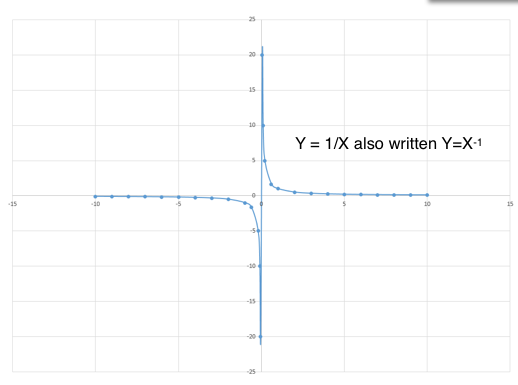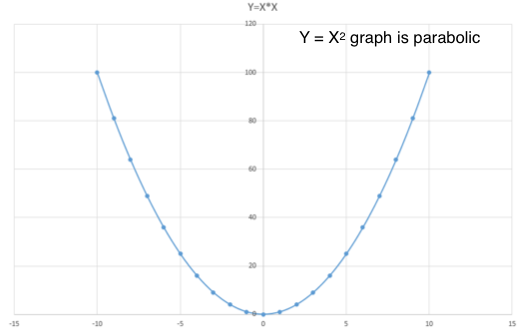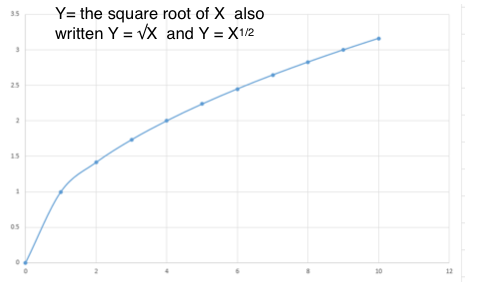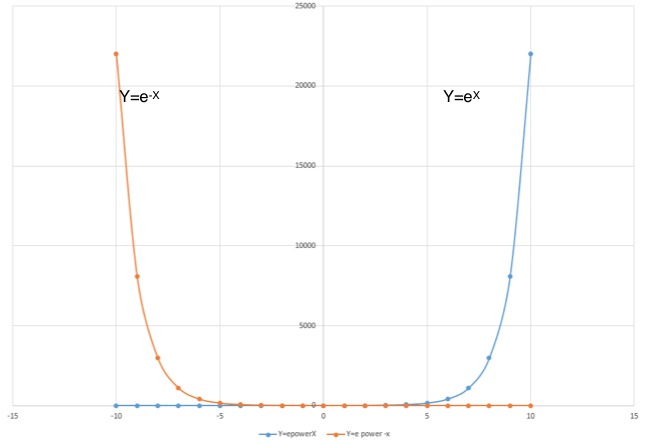### Y proportional to the inverse of XY proportional to the inverse of X is a common form of equations in physics and a curved graph like this will show the connection between the two. For example from the wave equation velocity = frequency x wavelength (V=fλ) then f is proportional to 1/λ. Since X can be either positive or negative Y can also be positive or negative

### Y proportional to X squared

The connection Y proportional to X squared is another common connection in physics equations. For example Kinetic energy = 1/2 mass x velocity squared (KE= 1/2mv2) and Einsteins famous equation E=mc2

Y will be always be positive even when X is negative because the square of a negative number is positive.### Y proportional to root X

Y proportional or equal to the square root of X  also written Y = √X  and Y = X1/2

Equations of this form are also common in physics, for example, velocity is proportional to the square root of kinetic energy   √2 KE/m = v

Since it is not possible to have a negative value of a square root both X and Y are always positive.### Exponential graphs in physics

Exponential graphs are important in several aspects of science for example in expanding populations, in the charge and discharge of capacitors and in radioactive decay.Other pages related to graphs and general physics ideas are: